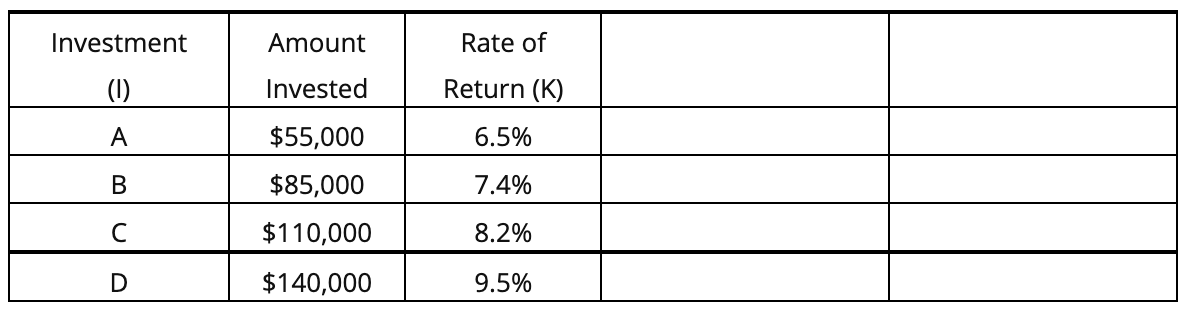# Question You have below a table on the amount of investment and the average Rate of Return on a portfolio of investments. Using the information in the table, prepare a table showing: The share of the total asset for each investment (A, B, C & D) What is the weighted average Rate of Return of the portfolio of investments? Investment Amount Rate of (1) Invested Return (K) A $55,000 6.5% B 7.4%$85,000 $110,000 C 8.2% D$140,000 9.5%Transcribed Image Text: Investment Amount Rate of (1) Invested Return (K) A $55,000 6.5% B 7.4%$85,000 $110,000 C 8.2% D$140,000 9.5%
Transcribed Image Text: Investment Amount Rate of (1) Invested Return (K) A $55,000 6.5% B 7.4%$85,000 $110,000 C 8.2% D$140,000 9.5%